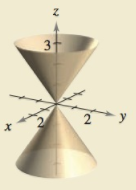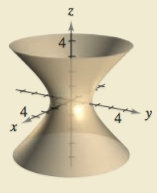Chapter 11.7, Problem 82E

Chapter
Section
Textbook Problem

# HOW DO YOU SEE IT? Identify the surface graphed and match the graph with its rectangular equation. Then find an equation in cylindrical coordinates for the equation given in rectangular coordinates.(a)(b)(i) x 2 + y 2 = 4 9 z 2 (ii) x 2 + y 2 − z 2 = 2

(a)

To determine
For a given graph, match of the graph with the given equations,

(i)x2+y2=49z2(ii)x2+y2z2=2

and convert the rectangular equation to cylindrical equation.

Explanation

Given:

The following provided graph is:

Explanation:

Conical graph is obtained for equations of the type x2+y2=cz2

Given graph is a cone.

So above graph matches the equation,

(i)x2+y2=49z2

Rectangular coordinates to cylindrical coordinates,

x2+y2=r2<

(b)

To determine
The match of the graph with the given equations,

(i)x2+y2=49z2(ii)x2+y2z2=2

and to convert the rectangular equation to cylindrical equation.

### Still sussing out bartleby?

Check out a sample textbook solution.

See a sample solution

#### The Solution to Your Study Problems

Bartleby provides explanations to thousands of textbook problems written by our experts, many with advanced degrees!

Get Started